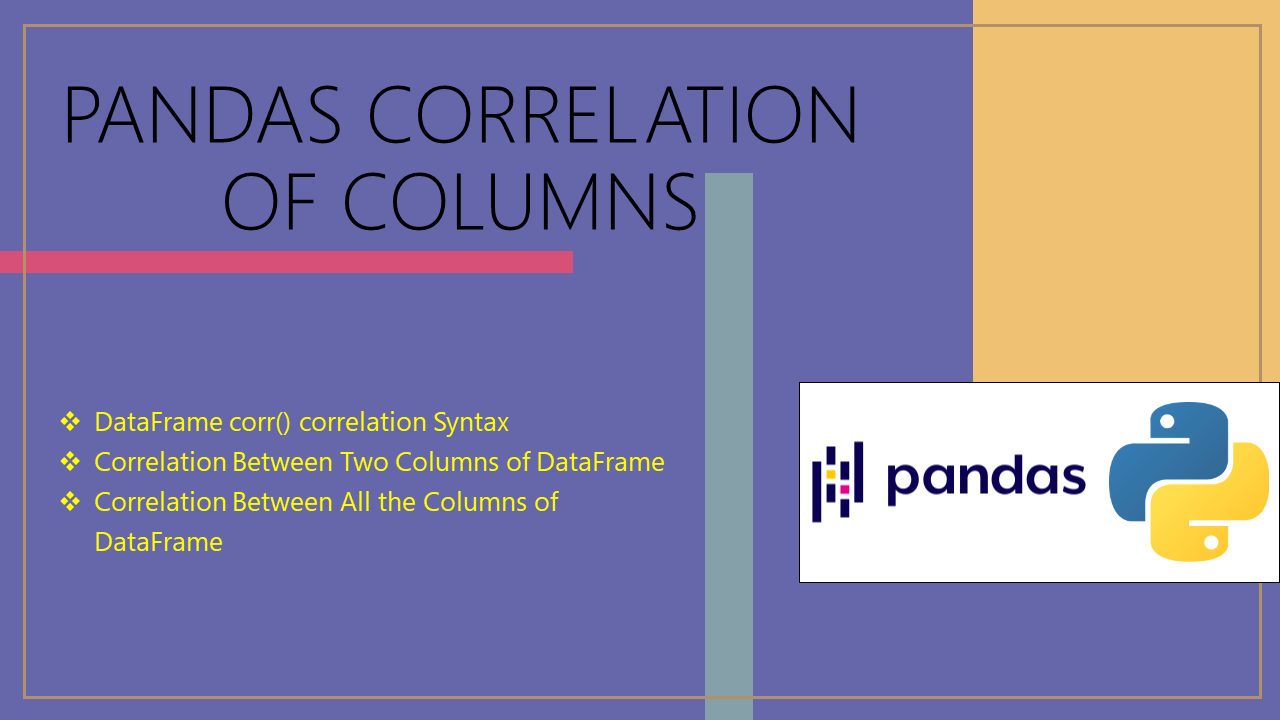# Pandas Correlation of Columns

• Post author:
• Post category:Pandas

pandas.DataFrame.corr() function can be used to get the correlation between two or more columns in DataFrame. Correlation is used to analyze the strength and direction between two quantitative variables. It is denoted by r and values between -1 and +1. A positive value for r indicates a positive association and a negative value for r indicates a negative association

In this article, I will explain how to get the correlation between two columns with several examples.

## 1. Quick Examples of Correlation of Columns

If you are in hurry below are some quick examples of pandas correlation between two columns.

``````
# Below are some quick examples.

# Correlation between two columns of DataFrame.
corr=df['Fee'].corr(df['Discount'])

# Correlation between all the columns of DataFrame.
df2=df.corr()

# Other example.
df['Discount']=np.float64(df['Fee'])
``````

Now, let’s create a pandas DataFrame with a few rows and columns, execute these examples and validate results. Our DataFrame contains column names `Courses``Fee` and `Duration`.

``````
# Create a pandas DataFrame.
import pandas as pd
import numpy as np
technologies= {
'Courses':["Spark","PySpark","Spark","Python","PySpark"],
'Fee' :[22000,25000,23000,24000,26000],
'Discount':[1500,1000,1200,800,1300],
'Duration':['30days','50days','30days','35days','40days']
}
df = pd.DataFrame(technologies)
print(df)
``````

Yields below output.

``````
# Output:
Courses    Fee  Discount Duration
0    Spark  22000      1500   30days
1  PySpark  25000      1000   50days
2    Spark  23000      1200   30days
3   Python  24000       800   35days
4  PySpark  26000      1300   40days
``````

## 2. DataFrame corr() correlation Syntax

Following is the syntax of the DataFrame.corr() function.

``````
# Syntax of corre()
DataFrame.corr(method='pearson', min_periods=1)
``````

## 3. Correlation Between Two Columns of DataFrame

You can see the correlation between two columns of pandas DataFrame by using `DataFrame.corr()` function. The `pandas.DataFrame.corr()` is used to find the pairwise correlation of all columns in the DataFrame. For example, let’s see what is the correlation between `Fee` and `Discount`.

``````
# Correlation between two columns of DataFrame.
corr=df['Fee'].corr(df['Discount'])
print(corr)
``````

Yields below output.

``````
# Output:
-0.35112344158839165
``````

We get -0.35 as the correlation between the scores of Fee and Discount. This indicates that the two columns highly correlated in a negative direction.

## 4. Correlation Between All the Columns of DataFrame

You can also get the correlation between all the columns of a pandas DataFrame. For this, apply `corr()` function on the entire DataFrame which will result in a DataFrame of pair-wise correlation values between all the columns.

Note that by default, the `corr()` function returns Pearson’s correlation.

``````
# Correlation between all the columns of DataFrame.
df2=df.corr()
print(df2)
``````

Yields below output.

``````
# Output:
Fee  Discount
Fee       1.000000 -0.351123
Discount -0.351123  1.000000
``````

When applied to an entire DataFrame, the `corr()` function returns a DataFrame of pair-wise correlation between the columns. We can see that there’s a weak negative correlation between scores of Fee/Discount. Also, notice that the values on the diagonal are 1s, this is because each column is perfectly correlated with itself.

## 5. Other Example

In this example, if Fee is float type, python skips it by default. All the other columns of DataFrame are in numpy-formats. so, you can do it by converting the column to `np.float64`.

``````
# Other example.
df['Fee']=np.float64(df['Fee'])
print(df)
``````

## 6. Complete Examples of Correlation Between Two Columns

``````
import pandas as pd
import numpy as np
technologies= {
'Courses':["Spark","PySpark","Spark","Python","PySpark"],
'Fee' :[22000,25000,23000,24000,26000],
'Discount':[1500,1000,1200,800,1300],
'Duration':['30days','50days','30days','35days','40days']
}
df = pd.DataFrame(technologies)
print(df)

# Correlation between two columns of DataFrame.
corr=df['Fee'].corr(df['Discount'])
print(corr)

# Correlation between all the columns of DataFrame.
df2=df.corr()
print(df2)

# Other example.
df['Fee']=np.float64(df['Fee'])
df2=df.corr()
``````

## Conclusion

In this article, you have learned how to get the correlation between two columns by using DataFrame.corr() method which can get positive and negative values between columns with several examples.

## References